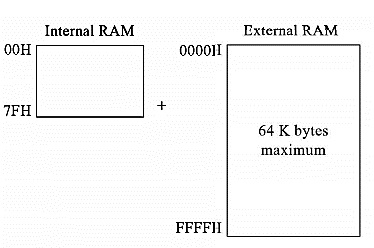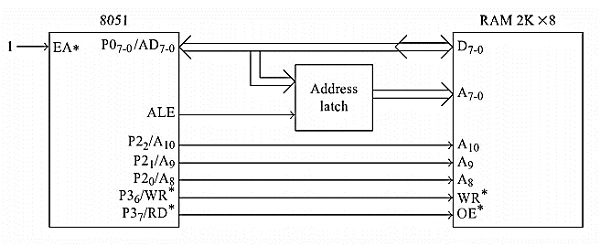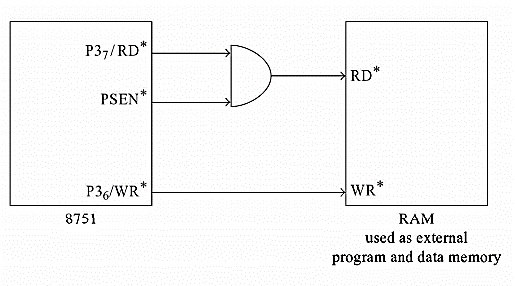# Data Memory Structure of Intel 8051

The 8051 has 128 bytes of On-Chip RAM. So for accessing that RAM area, the address space is 00H to 7FH. When we need more data memory, we can use external RAM. The address space of external RAM is 0000H to FFFFH.The external and internal data memory can be added to increase the total amount of data memory. When we are trying to access the external data memory, then the read RD or write WR will be the output from 8051. The external data memory address can be either 8-bit or 16-bit wide. Generally, the one 8-bit address is used but by using the one-byte address, only 256 bytes of memory can be addressed. So when we need a little bit of extra memory, we can use one-byte addresses by the Port 0 pins

When we need a little more space than 256 bytes, we can use a few lines of Port 2 pins. Let us consider a case, where three lines of Port 2 are used to select a page of 256 bytes. Remaining pins can be used for IO tasks. It is assumed that there is no external program memory. There are some instructions like MOVX A, @R1, here only one-byte data is sent out from Port 0.When we want to use large amounts of external data memory, we have to use instructions like MOVXA, @DPTR. By connecting the RD and PSEN of the 8051 to AND gate we can combine the data memory and program memory. By this scheme the data and program may overlap, so the programmer needs to be cautious about that